## Table of ContentOpen Access

ARTICLE

# Analysis of Temperature Rise Characteristics and Fatigue Damage Degree of ACSR Broken Strand

Jun Zhang1, Xiaobin Li1, Long Zhao2,*, Zixin Li1, Shuo Wang1, Pan Yao1, Pengfei Dai2

1 Jiangmen Power Supply Bureau, Guangdong Power Grid Co., Ltd., Jiangmen, 529030, China
2 School of electronic information, Xi’an Polytechnic University, Xi’an, 710048, China

* Corresponding Author: Long Zhao. Email:Energy Engineering 2023, 120(3), 617-631. https://doi.org/10.32604/ee.2022.024855

## Abstract

In this paper, the research on ACSR temperature of broken strand and fatigue damage after broken strand is carried out. Conduct modeling and Analysis on the conductor through Ansoft Maxwell software. The distribution of magnetic force lines in the cross section of the conductor after strand breaking and the temperature change law of the conductor with the number of broken strands are analyzed. A model based on electromagnetic theory is established to analyze the distribution of magnetic lines of force in the cross section of the conductor after strand breaking and the temperature variation law of the conductor with the number of broken strands. The finite element analysis results show that with the increase in the number of broken strands, the cross-sectional area of the conductor decreases, the magnetic line of force of the inner conductor at the broken strand becomes denser and denser, and the electromagnetic loss of the conductor becomes larger and larger. Therefore, the temperature of the conductor at the broken strand becomes higher and higher. Then, the current carrying experiment of conductor is carried out for LGJ-240/30 conductor. It is found that the temperature rise at the junction of inner and outer layers at the broken strand is particularly obvious, and the temperature of inner aluminum conductor at the broken strand also increases with the increase of broken strand. According to the analysis of experimental data, with the increase of broken strands, the antivibration ability and service life of the conductor decrease. At the same time, under certain conditions of broken strand, the fatigue life of conductor increases with the increase of temperature.

## Keywords

1  Introduction

In the context of the development of power system to new power system, the transmission capacity of China’s power grid is also facing a huge test . In the actual operation of the conductor, the conductor bears the influence of the natural environment, endangering the operation safety of the transmission line, and then affecting the operation safety of the whole power system .

The research on the fatigue life of conductor began in 1921. Most scholars pay attention to the aeolian vibration under the excitation of wind. Some experiments and phenomena also show that the aeolian vibration seriously threatens the safe operation of the line [3,4]. This is due to the action of aeolian vibration. On the one hand, the up and down vibration of the conductor makes the strands squeeze each other and slip and deform, resulting in fretting wear at the contact parts between the strands, and then a large number of cracks . On the other hand, fatigue damage caused by aeolian vibration also threatens the safety of transmission lines . In addition, the inclusions in the production process of the conductor are also easy to fracture the conductor strands under the action of vibration .

Scholars at home and abroad have done a lot of research on the fatigue life of transmission lines. As early as 1945, Miner  put forward the linear fatigue cumulative damage theory, which was affirmed by the international power grid conference in 1979. The conference recommended this theory as the basic theory for predicting the fatigue life of transmission conductors. Brunair et al.  verified the fatigue characteristics of ACSR under constant and variable amplitude loads by using the indoor wind-induced vibration simulation method. By comparing the experimental results with miner’s cumulative damage hypothesis, it is proved that miner’s cumulative damage hypothesis is somewhat conservative, but it can be assumed as an effective design purpose. Zhou et al.  simulated the stress state at the wire clamp in the laboratory and calculated the fatigue life of the wire from the local stress. Sinha’s research focuses on the connection of transmission line. The influencing factors of conductor fatigue life are studied by using local stress evaluation method . Wang et al.  put forward the fatigue test method and life estimation method under the action of composite alternating stress of transmission conductor. After that, Kong et al.  conducted wind tunnel experiments on Aeolian Vibration of transmission lines in combination with Miner theory, deduced the vibration model of dynamic method, and finally obtained the fatigue life prediction model of aeolian vibration of transmission lines.

However, the fatigue life of the conductor is not only related to vibration, but also related to the temperature of the conductor. The fatigue life is related to the stress of the conductor, and the temperature change of the conductor will cause the stress change, which is also confirmed . However, the conductor temperature is not only related to the current, but also related to the degree of damage. In particular, the cross-section current density increases after strand breaking. Under the joint action of skin effect, convective heat transfer and other phenomena, the temperature distribution changes. The change of temperature will further cause the change of parameters such as tensile strength and fatigue strength of the conductor, thus affecting the calculation of fatigue life. Under the action of tensile stress, the change of temperature will increase the creep of the conductor, and the further aggravated creep will cause material fatigue and fracture . But according to the research, the stiffness of all aluminum conductor does not change in a certain range of temperature .

In view of this phenomenon, the temperature rise characteristics and fatigue damage degree of ACSR after strand breaking are studied in this paper. Firstly, through Maxwell electromagnetic simulation, the distribution of magnetic lines of force in the cross-section of the conductor before and after strand breaking is studied. As the electric field intensity is directly proportional to the density of the magnetic lines of force, the denser the distribution of the magnetic lines of force, the greater the electromagnetic loss at the corresponding position, so as to judge the variation trend of the conductor section with the temperature of the broken strand. The current carrying experiment of LGJ-240/30 ACSR was established to study the temperature rise characteristics at different positions when the conductor was broken. Finally, according to the cumulative damage principle of transmission conductors recommended by CIGRE, the change law of conductor fatigue damage under certain amplitude wind vibration is discussed.

2  Simulation Analysis of Magnetic Field after Conductor Strand Breaking

2.1 Simulation Model of Electromagnetic Field Inside Conductor

In order to explore the variation law of the temperature of the conductor section with the number of broken strands, the conductor current carrying experiment is carried out for LGJ-240/30 ACSR, and a two-dimensional finite element model of the conductor section based on LGJ-240/30 is established.

In order to facilitate the calculation of the distribution of magnetic lines of force inside the conductor, the simulation makes the following assumptions: (1) Assume that the conductor electromagnetic field is a stable field under power frequency, ignoring the displacement current; (2) It is assumed that the permeability and conductivity in the conductor material are uniform, linear and isotropic; (3) Ignoring the kink characteristics of strands.

According to the above assumptions and the differential form of Maxwell’s equations, the vector magnetic potential A is introduced to list the governing equations of eddy current distribution in air domain and conductor domain of ACSR .

μ2Azr2+μr2Azθ2=Jz=γAztJzs(1)

where: μ is the permeability of conductor material, γ is the conductivity of conductor material, Az is the z-axial component of vector magnetic potential, r is the polar diameter component of polar coordinate, θ is the polar angle of polar coordinate, Jz is the z-axis component of current density, Jz is the z-axial component of power supply current density, t is time.

The steel core in the middle of the steel core aluminum strand is ferromagnetic material, and the relative permeability of aluminum is approximately 1. Therefore, the basic partial differential equation should be introduced for the boundary conditions of the interface of different media areas .

{Az1=Az2μ1Az1n=μ2Az2n(2)

where: Az1 is the vector magnetic potential z-axis component of the finite element surface in the medium 1 region, Az2 is the vector magnetic potential z-axis component of the finite element surface in the medium 2 region, μ1 is the permeability of the medium 1 region, μ2 is the permeability of the medium 2 region, n is the normal vector of the finite element boundary.

The heat source of the transmission conductor in the current carrying state mainly comes from the electromagnetic loss of the conductor. According to the differential form of Joule’s law, the thermal power density at any point in the conductor is directly proportional to the square of the electric field intensity at that point, and the proportional coefficient is the conductivity of the conductor at that point , as shown in formula (3):

P=VγE2dV(3)

where: E is the electric field strength, γ is the conductivity of the conductor material, dV is the unit volume.

In addition, the conductivity of the conductor material is determined by Eq. (4):

γ=γ201+α(T20C)(4)

where: γ20 refers to the conductivity at 20°C, α refers to the temperature coefficient of conductor conductivity varying with temperature, T refers to conductor temperature.

2.2 Magnetic Field Simulation Results and Analysis of Conductor Section

In this paper, a two-dimensional finite element model of conductor section is established for LGJ-240/30 ACSR. Ansoft Maxwell software is used to simulate and analyze its internal magnetic force line distribution. The simulation excitation is AC with frequency of 50 Hz and amplitude of 500 A. The simulation results are shown in Figs. 1 to 2.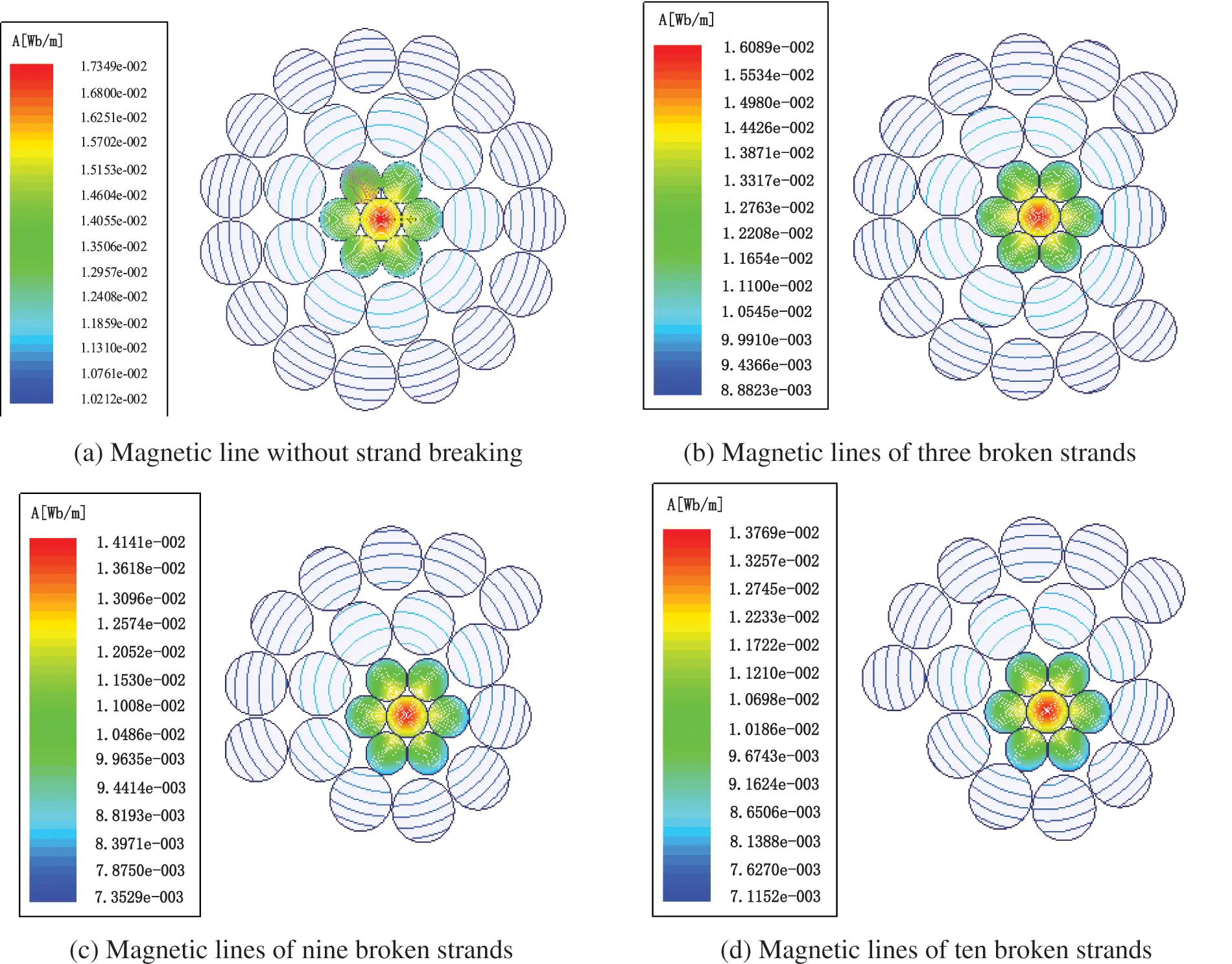Figure 1: Distribution of magnetic lines of force of conductors under different conditions

It can be seen from the figure that when the conductor is intact, the density of the magnetic lines of the outer aluminum wire is greater than that of the inner. Therefore, the eddy current loss of the outer aluminum wire is large. In addition, due to the skin effect of the conductor, the current density of the outer aluminum wire is greater than that of the inner aluminum wire, so the ohmic loss of the outer conductor is greater than that of the inner aluminum wire. However, in practice, the comprehensive conditions such as conductive heat generation, sunshine heat absorption and natural convection heat dissipation of the conductor should be considered. In view of the broken strand of the conductor, the density of magnetic lines of force of the inner aluminum wire at the broken strand increases, the eddy current loss increases, the broken strand leads to the current density of the conductor cross-sectional area increases, the direct current resistance of the conductor increases, and the ohmic loss increases.

3  Experimental Environment

To explore the temperature change’s law while strand broke, the conductor current carrying experiment environment is established. After changing the number of broken strands of ACSR by external force, the current carrying experiment is carried out on the broken strand conductor. During the experiment, the temperature value of the conductor is monitored and analyzed. The experimental platform is shown in Fig. 2.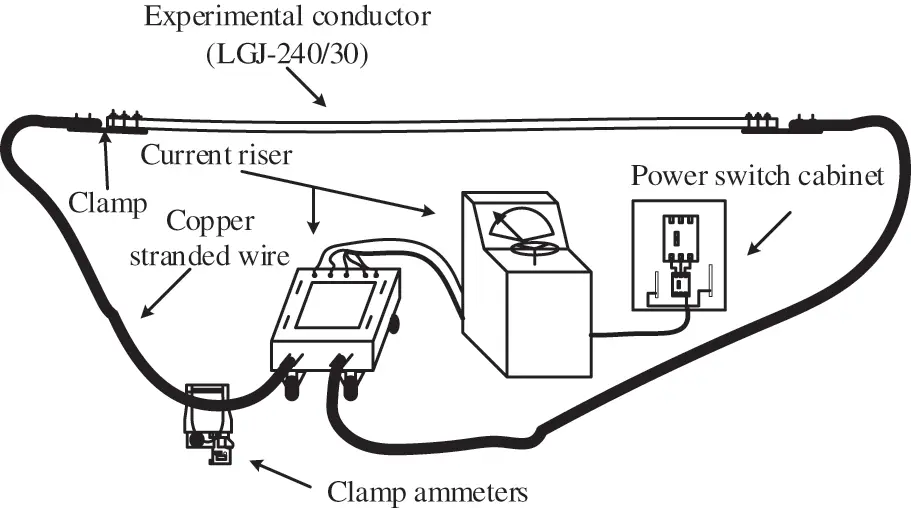Figure 2: Experimental environment

The experimental platform is in a closed environment. The experimental device mainly includes adjustable current riser, LGJ-240/30 ACSR, infrared thermal imager, clamp ammeter and copper strand. The current riser can output 1~1000 A AC. Infrared thermal imager is used for measuring temperature. The visual temperature difference of thermal sensitivity (NETD) is less than 0.12°C. The current lifter raises the energizing current to the current required for the experiment. The time for each strand breaking current carrying test is sufficient to ensure that the temperature of the conductor can reach a steady state under the condition of current carrying. In addition, the ambient temperature remained constant during the experiment.

The model of ACSR selected in the experiment is LGJ-240/30 conductor as the experimental object, and the geometric parameters of the conductor are shown in Table 1.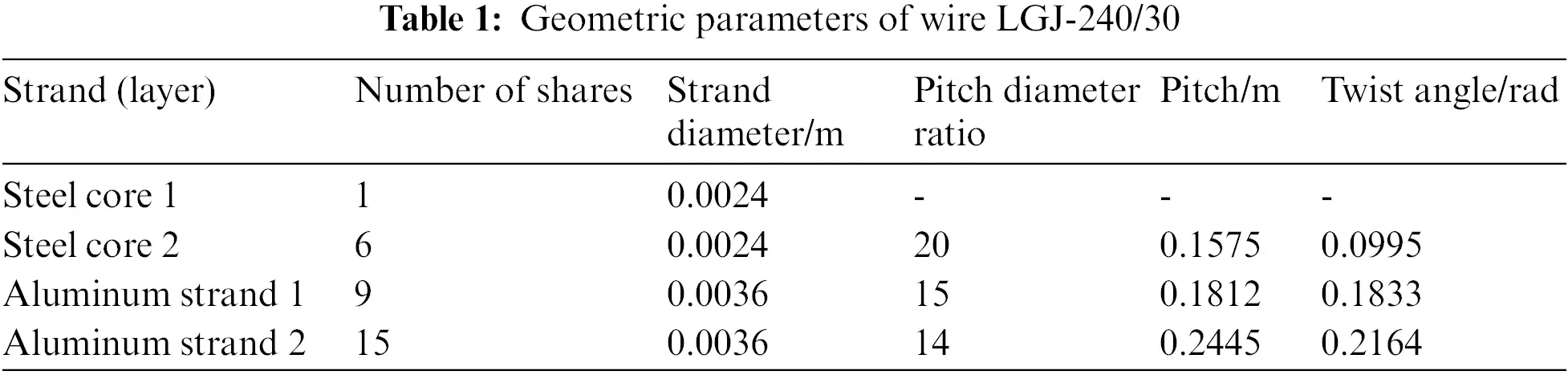Use the infrared imager to measure the wire temperature, and mark the places where the wire needs to be measured according to the experimental needs. The marking points include the left clamp, the right clamp, the marking point 1, the fault marking point (the marking points after strand breaking are the local temperature of strand breaking, the temperature of intermediate aluminum wire and the temperature of strand around strand breaking), and the marking point 2. The marking points are shown in Fig. 3. The time of each conductor current carrying test is 70 min to make the conductor temperature reach a steady state. The infrared thermal imager is used to measure the conductor temperature data every 5 min. Fig. 4 is an infrared thermal imaging diagram of a broken strand position taken using infrared thermal imaging.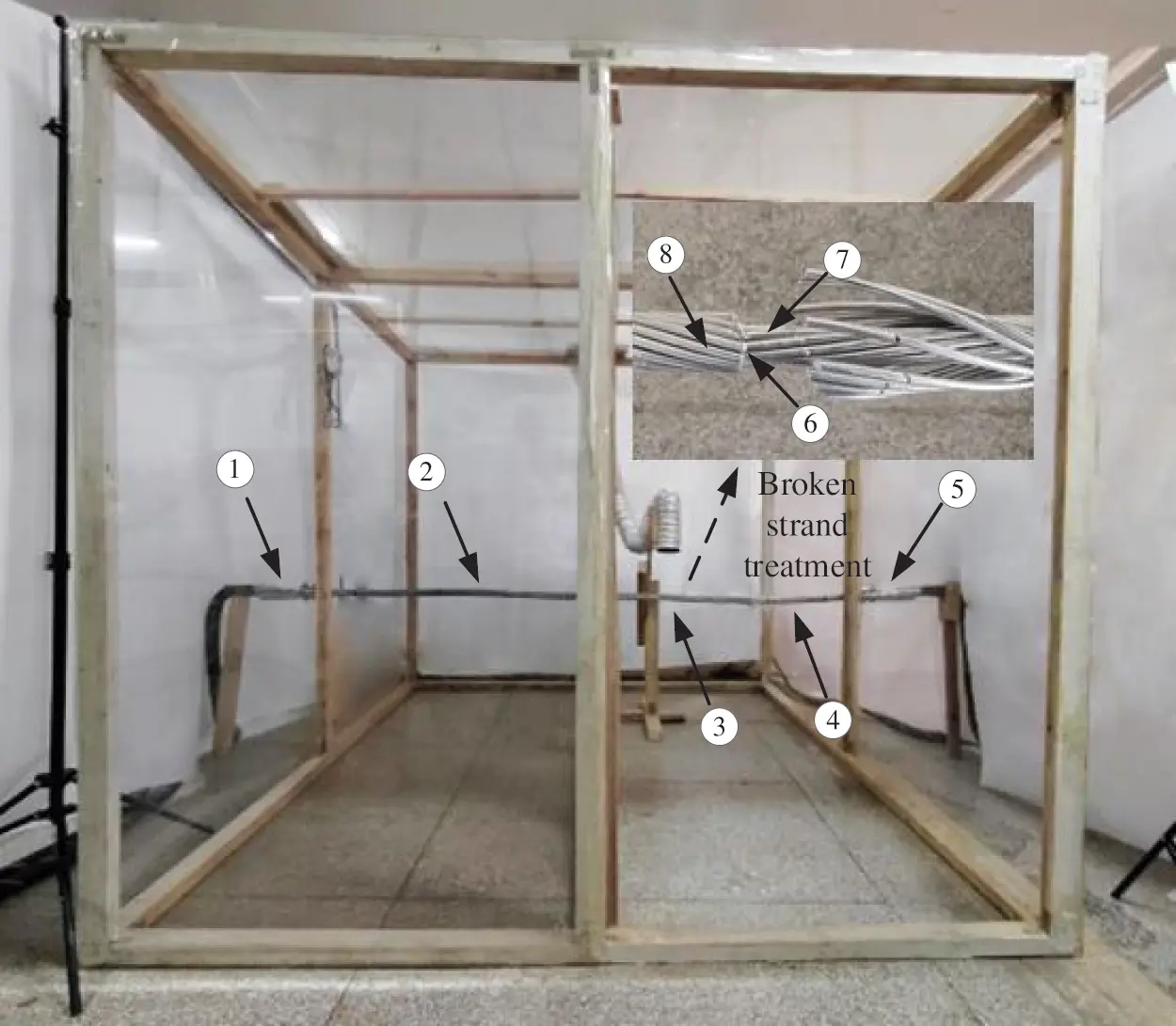Figure 3: Wire temperature measurement points. ①-left clamp, ②-marking point 1, ③-fault marking point, ④-marking point 2, ⑤-right clamp, ⑥-junction of inner and outer layers of broken strand, ⑦-middle aluminum wire of broken strand, ⑧-outer aluminum wire around broken strand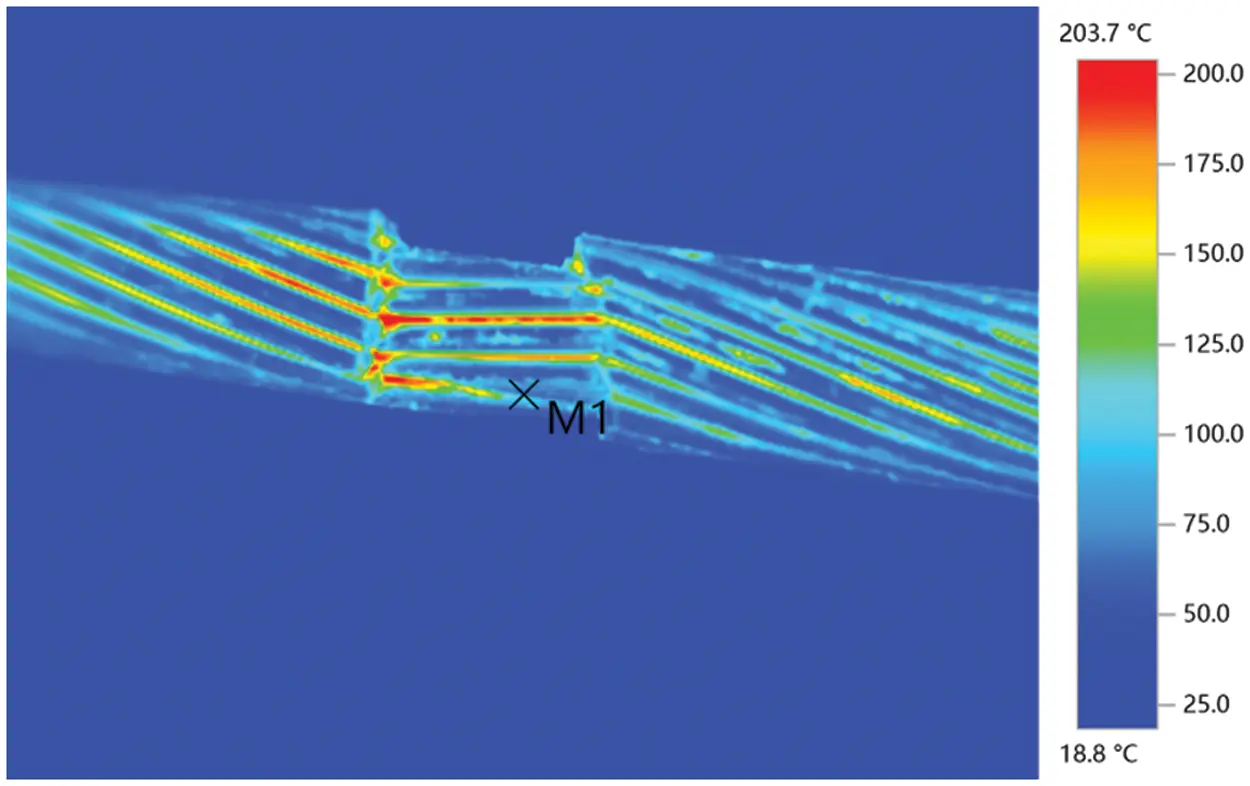Figure 4: Infrared thermogram

4  Experimental Results and Analysis

4.1 Experimental Process

According to the rated current limit of LGJ/240-30 conductor, set the experimental energizing current range as 100 A--500 A and the step size as 100 A. Record the data of monitoring points every 5 min and a group of 70 min. The emissivity of alumina is 0.4, and the emissivity of conductor surface is subject to the oxidized aluminum. The following Fig. 5 the temperature data of the conductor and clamp after reaching the steady state.Figure 5: Temperature change curve

4.2 Analysis of Temperature Rise Characteristics

(1) Temperature rise characteristics at clamp

It can be seen from Fig. 5, the conductor temperature at the left and right clamps (① and ⑤) is significantly higher than that at the left clamp (①), and the conductor temperature at the right clamp (⑤) is also slightly higher than that at the left clamp (①). The main reason for this phenomenon is the poor contact area between the conductor and the clamp. At the same time, in the actual working conditions, Loose bolts at the clamp will also cause serious heating of the clamp, so it is very necessary to monitor the temperature at the clamp.

(2) Temperature rise characteristics of outer aluminum wire around broken strand

It can be seen from Figs. 5 and 6 that the temperature of ⑧ is slightly higher than that of ②, and the temperature of both is significantly higher than that of the conductor without broken strand. While the temperature of ⑥ is higher than that of ⑧ and ②. Due to heat conduction, the temperature of ② will be significantly higher than that of the conductor when the strand is not broken. Due to the reduction of the cross-sectional area of the conductor, the current density of the outer aluminum wire (⑧) around the broken strand increases, resulting in the increase of conductor heating. In addition, the heat conduction close to the temperature at ⑥, so the temperature will be higher than the temperature at ②.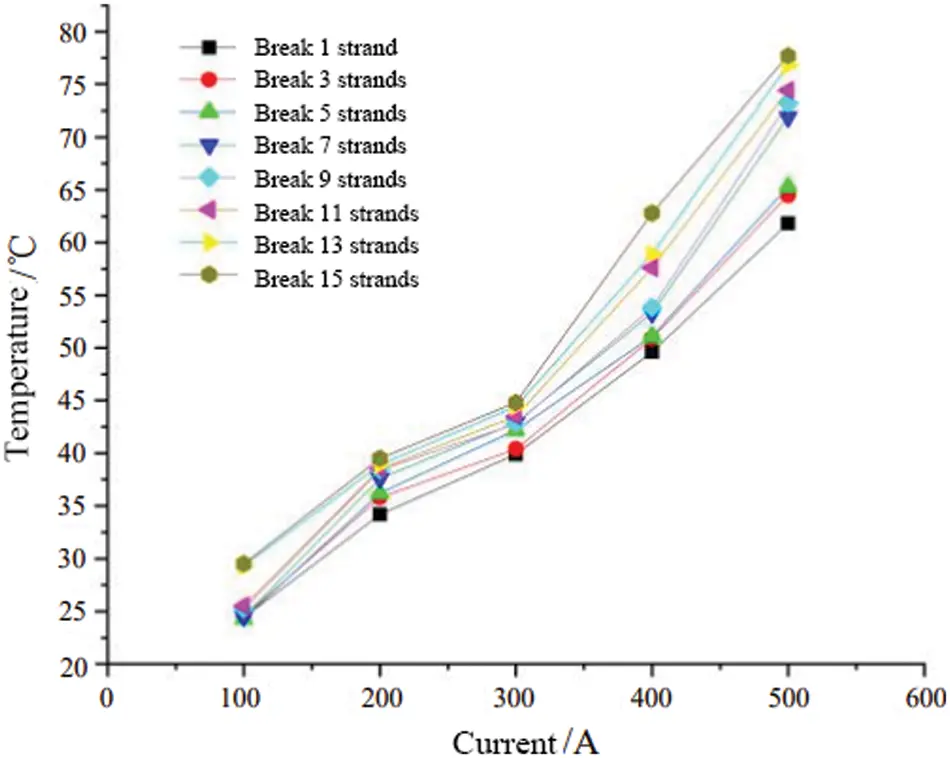Figure 6: Temperature of outer aluminum wire around broken strand

(3) Temperature rise characteristics of intermediate aluminum wire

It can be seen from Figs. 6 and 7, the temperature of the intermediate aluminum wire (⑦) at the broken strand position is slightly higher than that of the outer aluminum wire (⑧) around the broken strand, and the temperature of the intermediate aluminum wire (⑦) will increase with the increase of broken strand and current carrying capacity. The main reason for this phenomenon is that the cross-sectional area of the conductor where the intermediate aluminum wire (⑦) is located decreases with the increase of the number of broken strands, resulting in the increase of current density and the increase of heat generation on this section.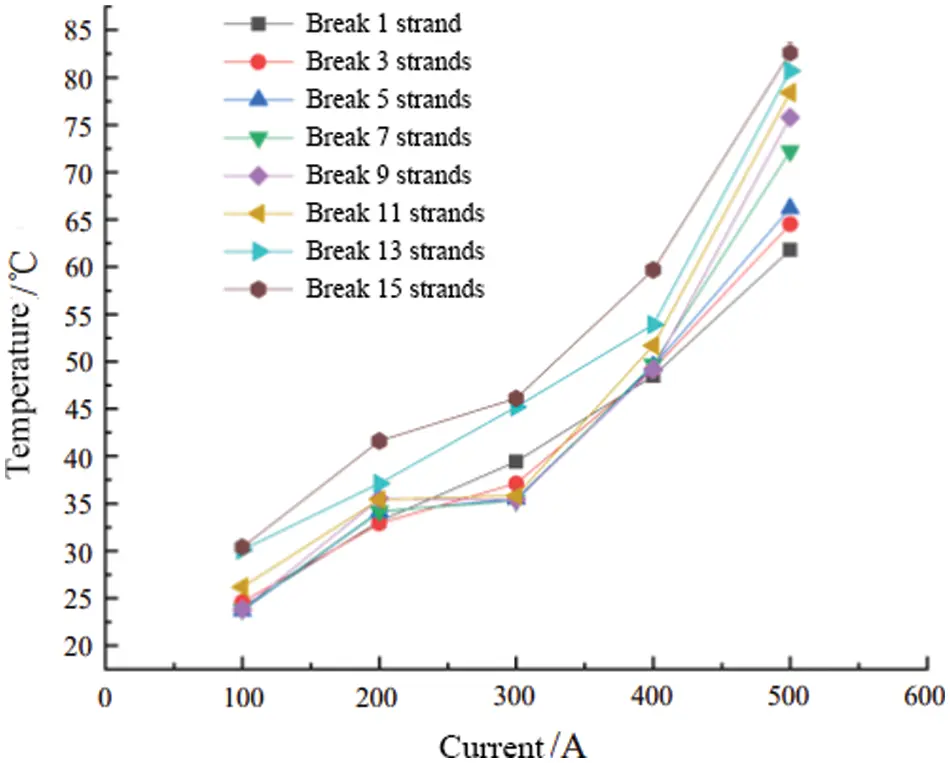Figure 7: Temperature of middle aluminum wire

(4) Temperature rise characteristics at the junction of inner and outer layers of broken strands

It can be seen from Figs. 7 and 8, the temperature at ⑥ is significantly higher than the temperature of ⑦ at the junction of broken strands and the temperature of ⑧. With the increase of current carrying capacity or the number of broken strands, the temperature at ⑥ will also increase significantly. When one strand is broken at a current carrying capacity of 500 A, The measured temperature at this place has exceeded the allowable temperature limit (70°C) of the conductor of the transmission line specified in the current design code of China, and the temperature increases with the increase of the number of broken strands. The main reason for this phenomenon is that the heat dissipation area at ⑥ is small, and with the increase of broken strands and current carrying capacity, the current density of conductor section will increase, which will increase the heat production of conductor at broken strands.Figure 8: Temperature at the junction of the inner and outer layers of the broken strand

4.3 Temperature Variation Curve of Broken Strand Conductor

It can be seen from Fig. 9 that the conductor temperature has reached steady state after 40 min of current carrying, so the data after 40 min can be used as steady-state data. The steady-state data with time nodes of 55, 60, 65 and 70 min under the current carrying experiment after conductor breaking are selected.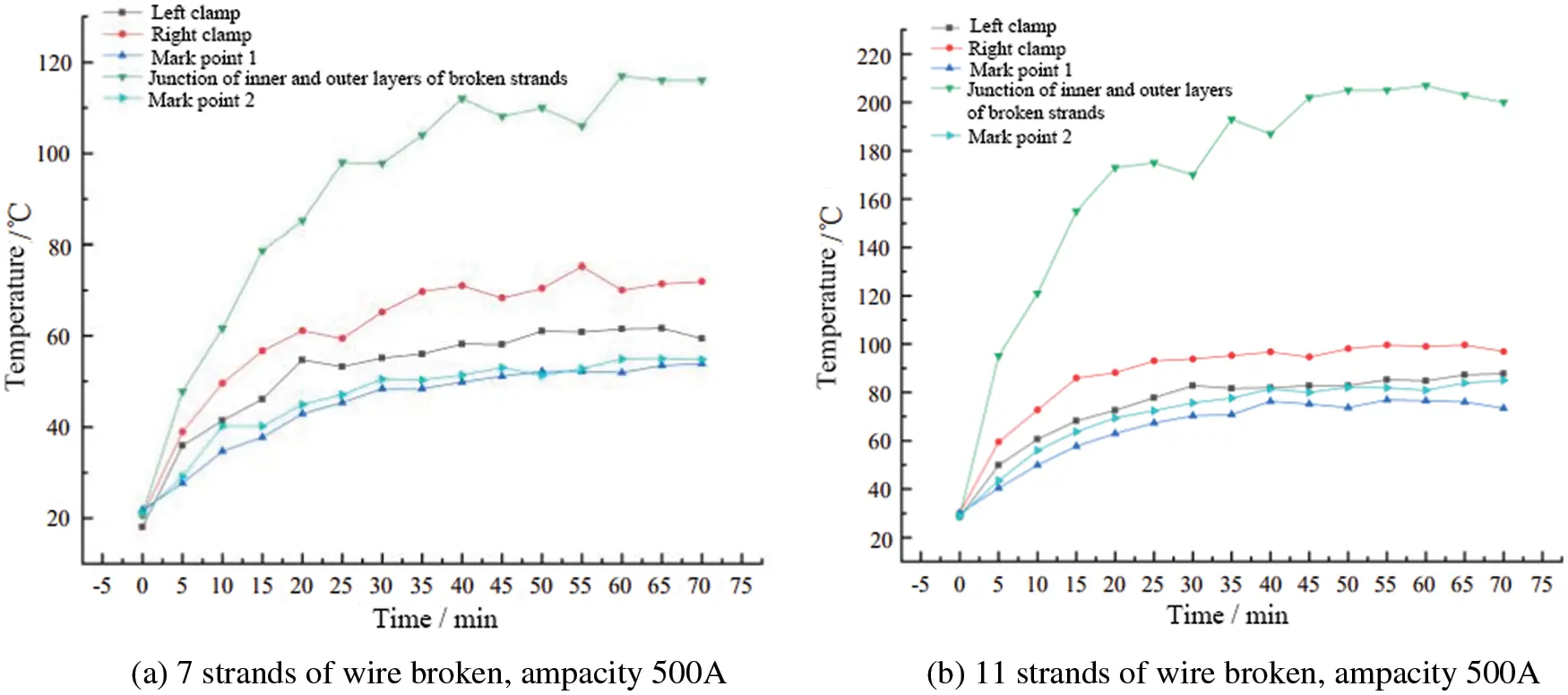Figure 9: Temperature rise characteristics

After the conductor is broken, the natural frequency of its vibration will decrease. According to literature , the health state of the conductor is judged according to the natural frequency of the conductor. When the natural frequency of the conductor changes, according to the recorded data, consider whether extreme weather has occurred and whether the change of natural frequency returns to the original value over time. If the natural frequency does not return to the original value after the change, it is determined that the structure has changed. Therefore, broken strands of wires can be monitored.

According to the four steady-state data collected from the experiment before and after the wire strand is broken, the proportional coefficient d is introduced to reflect the quotient difference of the temperature before and after the wire strand is broken, as shown in formula (5). Plot the obtained D, use linear data fitting, and briefly predict the temperature rise characteristics of the wire, as shown in Fig. 10.

d=TnT(5)

where, T is the temperature of the conductor when the strand is not broken, n is the number of broken strands, Tn is the temperature at the junction of the inner and outer layers of the broken strand.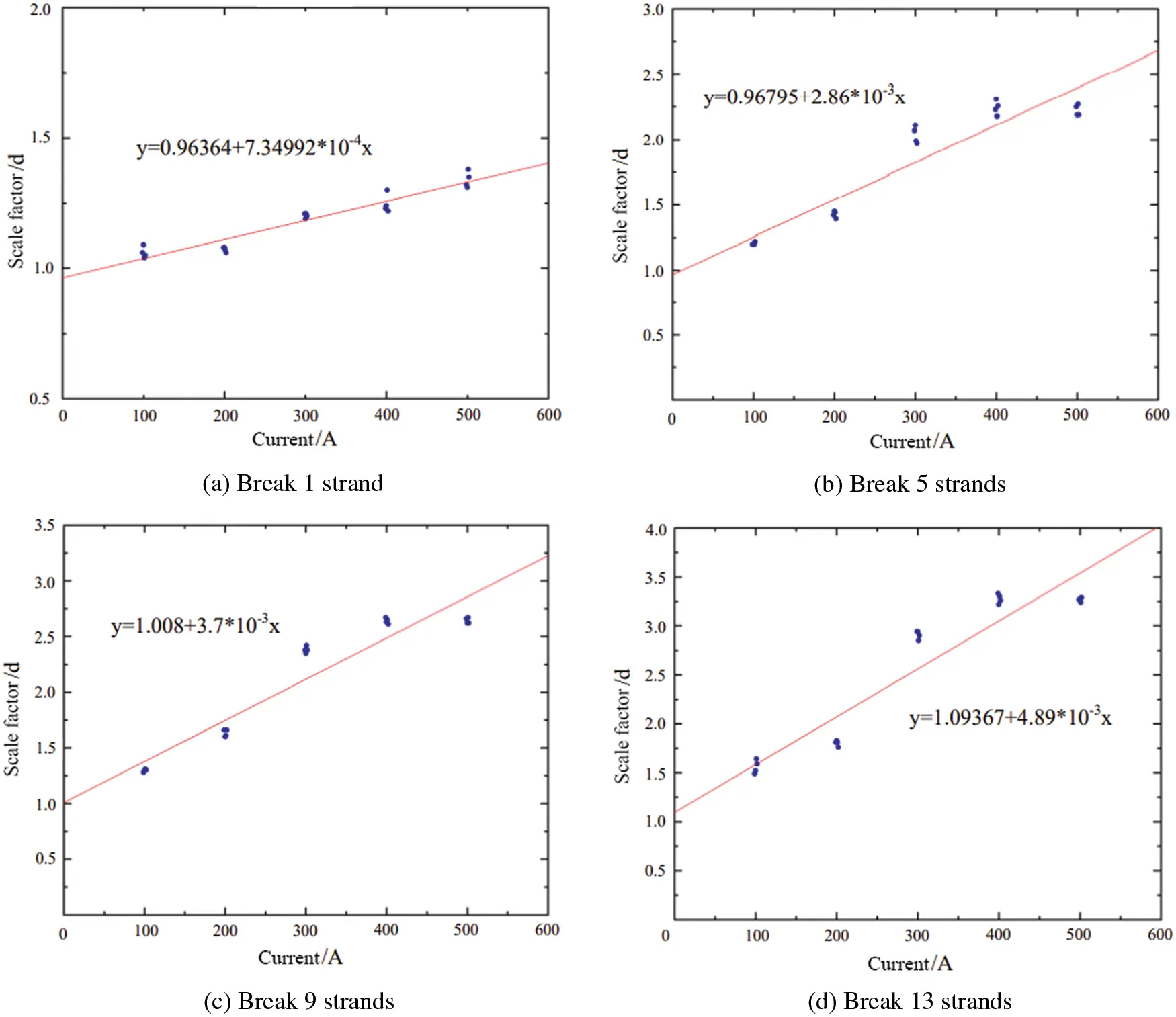Figure 10: Temperature proportional coefficient D-current curve of LGJ-240/30 wire

As shown in Fig. 10, the curve can reflect the general law of temperature change with current carrying capacity after strand breaking under ACSR current carrying conditions, and the curve distortion will become more serious with the increase of strand breaking. For a few strands, the curve has certain analysis value for the temperature change after strand breaking.

5  Analysis of Fatigue Damage Degree of Conductor Considering Current Action

5.1 Conductor Damage Principle

Fatigue life prediction is the most ideal method for transmission line damage identification . The dynamic bending strain is calculated by measuring the bending amplitude of the point on the conductor at the last contact point 0.089 m between the conductor and the clamp, so as to predict the fatigue life of the conductor .

K=Ki=i=1m(ni/Ni)(6)

Among them, ni is the actual vibration times under stress σi, the total vibration times causing fatigue damage under stress σi, Ki is fatigue damage of conductor under vibration stress σi. When K=1, the wire will break. Among them, the total vibration times of fatigue failure can be calculation calculated by σi, and under a certain amplitude, σi can be calculated according to Hooke’s Law:

σi=Ep2DYa4(epx1+px)(7)

where, E is the elastic modulus of outer strand, D is the radius of conductor, x=0.089m, Ya is the vibration amplitude, p is the bending stiffness parameter.

p=T/EI(8)

where, T is the tension, EI is the bending stiffness of the conductor, which can be calculated by Eqs. (9) and (10) :

T=lslsi=0n(zicos3αi1+visin2αiEiAi)(9)

where n is the number of layers of the conductor, n = 0 of the center line, ls is the length of the strand, ls is the elongation of the strand, αi is the twist angle of the strand in layer i, zi is the number of wires in layer i, and vi is the ratio of the circumferential transverse shrinkage and axial shrinkage of the spiral strand radius relative to the stretching of the transmission conductor.

EI=π64(nald4alEal+nstd4stEst)(10)

where nal is the number of aluminum strands, dal is the diameter of aluminum, Eal is the young’s modulus of elasticity of aluminum, nst is the number of steel cores, dst is the diameter of steel cores, and Est is the young’s modulus of elasticity of steel.

At present, it is customary to determine the number of conductor vibration that can cause conductor fatigue failure under a certain stress level according to the Wöhler safety boundary curve, that is, the value of N, and the expression is as follows :

{σi=450Ni0.2(Ni2×107)σi=263Ni0.168(Ni>2×107)(11)

It can be seen from formulas (5), (6) that there is a strong correlation between fatigue damage and elastic modulus under the same amplitude.

E=E0(125at)(12)

where t is the temperature and a is the coefficient.

5.2 Conductor Damage Analysis

According to formula (6), when the vibration of the conductor reaches the total vibration times of fatigue failure, k = 1, the conductor will undergo fatigue fracture.

The tension T of the corresponding single strand of the transmission conductor increases with the increase of the broken strand of the conductor. At the same time, with the increase of the number of broken strands, it can be seen from (12) that the local temperature at the broken strand of the conductor will rise, resulting in the decrease of the elastic modulus of aluminum, but the decrease of the elastic modulus in the numerator of formula (7) and the decrease of the elastic modulus in the denominator of the square of formula (8) offset each other, and the increment of numerator in formula (7) is greater than that of denominator. Therefore, it can be judged that with the increase of conductor strand breaking, the tension of single strand of conductor increases, resulting in the increase of stress σi.

It can be seen from (11) that when the stress σi of the conductor increases. Under this stress, the number of conductor vibration Ni that can cause conductor fatigue failure will decrease. Therefore, it can be seen from formula (6) that when Ni decreases, the broken strand will be the first to break under the same number of wire vibrations as the unbroken wire.

When the wire strand is broken for a certain time, the numerator and denominator in formula (8) have elastic modulus, so the influence of elastic modulus on bending stiffness parameter p can be ignored. With the increase of conductor current carrying capacity, the conductor temperature will also rise. According to Eq. (12), the elastic modulus of the conductor decreases with the increase of the conductor temperature. Further, it can be seen from Eq. (7) that the conductor stress σi decreases.

It can be seen from (11) that when the stress σi on the conductor decreases. Under this stress, the number of conductor vibration Ni that can cause conductor fatigue failure will increase. Therefore, it can be seen from formula (6) that when Ni rises and the number of conductor vibration is the same as that of the unbroken conductor, the broken conductor will break later and the fatigue life of the conductor will increase. However, with the increase of temperature, the sag will increase, so it is very important to select the appropriate operating current.

6  Conclusion

Through the finite element analysis of the broken strand conductor, the magnetic field line distribution of the conductor section is obtained, and the temperature rise characteristics of the broken strand conductor are obtained by setting up the conductor current carrying experiment. The following conclusions are obtained:

(a) When the current carrying capacity of the conductor is the same, the cross-sectional area of the conductor decreases with the increase of the number of broken strands, resulting in the increase of the resistance of the aluminum conductor, and the density of magnetic lines at the broken strands is greater than that of the outer unbroken strands, so the temperature at the broken strands of the conductor increases with the increase of the number of broken strands.

(b) The experimental phenomena show that the temperature rise characteristics at the junction of the inner and outer layers of the broken strand are particularly obvious, and the temperature of the clamps on both sides under the same conditions is significantly higher than the conductor temperature. The temperature at the clamp increases with the increase of the current carrying capacity of the conductor and with the increase of the broken strand of the conductor.

(c) According to the proportional coefficient current equation, the slope of the equation increases with the increase of broken strands, and the temperature rise characteristics of the conductor become more obvious with the increase of broken strands.

(d) According to the analysis of the fatigue damage degree of the running conductor, with the increase of the broken strand, the tension and stress of the single strand conductor increase, which leads to the reduction of the vibration times of the conductor causing the fatigue failure of the conductor, and shortens the fatigue life of the conductor.

(e) According to the analysis of the fatigue theory of the running conductor, the temperature rise characteristic of the broken strand of the conductor will increase the fatigue life of the conductor, which is due to the effect of temperature change and the elastic modulus of the conductor, and the temperature rise at the local position of the broken strand is more obvious.

Funding Statement: This work is supported by Special Topic of Infrastructure Technology Innovation of Guangdong Power Grid Co., Ltd. (0307002021030103XG00163), and by Natural Science Basis Research Plan in Shaanxi Province of China (2022JQ-568).

Conflicts of Interest: The authors declare that they have no conflicts of interest to report regarding the present study.

## References

1. Hou, Y., Wang, W., Wei, H., Deng, X. J., & Ji, Q. H. (2021). Research and application of transmission line dynamic capacity enhancement technology. Automation of Electric Power Systems, 45(17), 189-198. [Google Scholar]
2. Azevedo, C. R. F., & Cescon, T. (2002). Failure analysis of aluminum cable steel reinforced (ACSR) conductor of the transmission line crossing the Parana River. Engineering Failure Analysis, 9(6), 645-664. [Google Scholar] [CrossRef]
3. Gomes, F. B. (2015). Análise comparativa de aparelhos para medição de vibração em cabos condutores de energia e cálculo da vida remanescente em cabos (Ph.D. Thesis). The University of Brasilia, Brazil.
4. Lévesque, F., Goudreau, S., Cardou, A., & Cloutier, L. (2010). Strain measurements on ACSR conductors during fatigue tests I—Experimental method and data. IEEE Transactions on Power Delivery, 25(4), 2825-2834. [Google Scholar] [CrossRef]
5. Chen, Y., Wang, Q. F., & Gu, L. L. (2004). Discussion on ultrahigh voltage wire and tower structure in China. High Voltage Engineering, 30(6), 38-41. [Google Scholar]
6. Sier, R. J., Riggio, J. C. (2003). Analysis of factors contributing to conductor failures on a 345 kV line. Transmission and Distribution Conference and Exposition, pp. 904–908. Dallas, USA.
7. Karabay, S., Ertürk, A. T., & Zeren, M. (2017). Failure analysis of wire-breaks in aluminum conductor production and investigation of early failure reasons for transmission lines. Engineering Failure Analysis, 83(1), 47-56. [Google Scholar]
8. Miner, M. A. (1945). Cumulative damage in fatigue. Journal of Applied Mechanics, 67, A–159. [Google Scholar]
9. Brunair, R. M., Ramey, G. E., & Duncan, R. R. (1988). An experimental evaluation of S–N, curves and validity of Miner’s cumulative damage hypothesis for an ACSR conductor. IEEE Transactions on Power Delivery, 3(3), 1131-1140. [Google Scholar] [CrossRef]
10. Zhou, Z. R., Goudreau, S., & Fiset, M. (1995). Single wire fretting fatigue tests for electrical conductor bending fatigue evaluation. Wear, 181–183(95), 537-543. [Google Scholar] [CrossRef]
11. Sinha, N. K., & Hagedom, P. (2007). Research and application of transmission line dynamic capacity enhancement technology. Journal of Sound & Vibration, 301(1), 400-409. [Google Scholar] [CrossRef]
12. Wang, J. C., & Xu, N. G. (2001). Test method for wire fatigue under compound alternating stress. Electric Power Constructio, 22(2), 18-20. [Google Scholar]
13. Kong, D. Y., Li, L., Long, X. O., & Ye, Z. X. (2010). Analysis of influencing factors of breezy vibration fatigue life of transmission line. Journal of Wuhan University of Technology, 32(10), 53-57. [Google Scholar]
14. Ning, P. (2021). Study on the fatigue life of overhead transmission wire under different temperature fields (Master Thesis). Northeast Electric Power University, Jilin, China.
15. Ertürk, A. T., Güven, E. A., & Karabay, S. (2015). Determination of Zr inoculation effect on improving thermal resistivity of EC grade aluminum. Transactions of the Indian Institute of Metals, 68(5), 535-541. [Google Scholar] [CrossRef]
16. Costa, E. R., Araújo, J. A., & Veloso, L. A. C. M. (2020). Development of controlled heating for fatigue test in overhead conductors at high temperature. Journal of the Brazilian Society of Mechanical Sciences and Engineering, 42(7), 1-14. [Google Scholar] [CrossRef]
17. Feng, C. Z., Ma, X. K. (2010). Introduction to engineering electromagnetic fields. Beijing, China: Higher Education Press.
18. Li, Y., Chen, Y., Wu, Z. K., Liu, S. W., & Liu, G. (2017). Overheating characteristics of steel–cored aluminum strand broken. Guangdong Electric Power, 30(6), 98-102. [Google Scholar]
19. Jia, Z. G., Xue, Q. Z. (2011). Electromagnetism. Beijing, China: Higher Education Press.
20. Zhao, L., Huang, X. B., Zhang, Y., Zhu, Y. C., & Jia, J. (2020). Aeolian vibration-based structural health monitoring system for transmission line conductors. Structural Control & Health Monitoring, 27(6), e2538. [Google Scholar] [CrossRef]
21. Karabay, S., Güven, E. A., & Ertürk, A. T. (2012). Performance testing of an optical ground wire composite. Materiali in Tehnologije, 47(1), 119-124. [Google Scholar]
22. Langlois, S., Legeron, F., & Levesque, F. (2014). Time history modeling of vibrations on overhead conductors with variable bending stiffness. IEEE Transactions on Power Delivery, 29(2), 607-614. [Google Scholar] [CrossRef]
23. Wang, H., Liu, Y. B., & Dong, Y. M. (2008). Study on vibration amplitude of overhead line fatigue test. Proceedings of the CSEE, 28(4), 123-128. [Google Scholar]
24. Li, P. S., Xiong, J., Liu, G. P., & Gao, C. (2020). Cross section stress analysis of overhead bare wire. Machinery Design and Manufacture, 2020(7), 146-149+155. [Google Scholar]

Zhang, J., Li, X., Zhao, L., Li, Z., Wang, S. et al. (2023). Analysis of Temperature Rise Characteristics and Fatigue Damage Degree of ACSR Broken Strand. Energy Engineering, 120(3), 617–631.This work is licensed under a Creative Commons Attribution 4.0 International License , which permits unrestricted use, distribution, and reproduction in any medium, provided the original work is properly cited.

View

Like

## Related articles

• F. Cianetti
• F. Curà, A. Gallinatti, R. Sesana
• L. Solazzi, C. Petrogalli, M....
• I. Černý, D. Mikulová